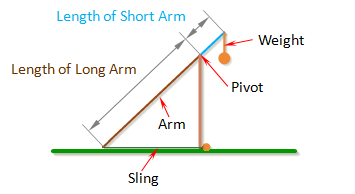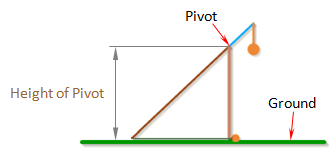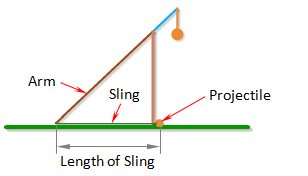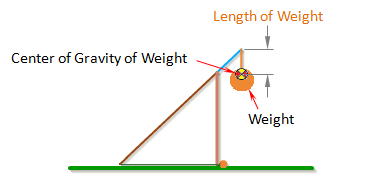Arm Lengths

The arm of the trebuchet is comprised of two sections: the long component of the arm which is the section that goes from the pivot point to the end where the sling attaches to the arm, and the short component which is the section that goes from the pivot to the end where the weight attaches.

The length of the long component of the arm is called Length of Long Arm.

The length of the short component of the arm is called Length of Short Arm.The optimal ratio between the long part of the arm and the short part of the arm will depend mainly on the Mass of Weight. If the weight is very heavy, the long part of the arm can be many times the length of the short part of the arm, which would allow the projectile to attain high velocities.

Height Of Pivot

Height of Pivot is the distance from the ground to the pivot.

The pivot is where the arm connects to the frame of the trebuchet, and the point about which the arm rotates.Note: To prevent the weight from hitting the ground, the height of the pivot should be greater than the sum of the Length of Short Arm and the total length of the weight.

Length of Sling

Length of Sling is the length of the sling that connects the projectile to the arm.In general, the trebuchet works best if the dimension Length of Sling is just a little less than the dimension Length of Long Arm.

Length of Weight

Length of Weight is the distance from the end of the arm to the center of gravity of the weight.Note: If Length of Weight is set to zero, the simulation uses a different set of equations that assume the weight is rigidly attached to the arm. This is how you simulate a fixed weight trebuchet.

 Units English (feet)English (inches)Metric Length of Short Arm ft Length of Long Arm ft Length of Sling ft Length of Weight ft Height of Pivot ft Uniform arm Mass of Arm lb Inertia of Arm lb·ft² Pivot to Arm CG ft Mass of Weight lb Inertia of Weight lb·ft² Projectile BaseballGolf BallPumpkinCowCustom Mass of Projectile lb Projectile Diameter ft Wind Speed ft/s Release Angle ° Play speed: 1x Link to save this trebuchet

VirtualTrebuchet 2.0

VirtualTrebuchet is a web based trebuchet simulator that will allow you to quickly evaluate different trebuchet configurations.

1. To begin, enter the parameters of your trebuchet in the input boxes.
2. Next, press the "Simulate" button located under the inputs.
3. Finally, watch your trebuchet go.